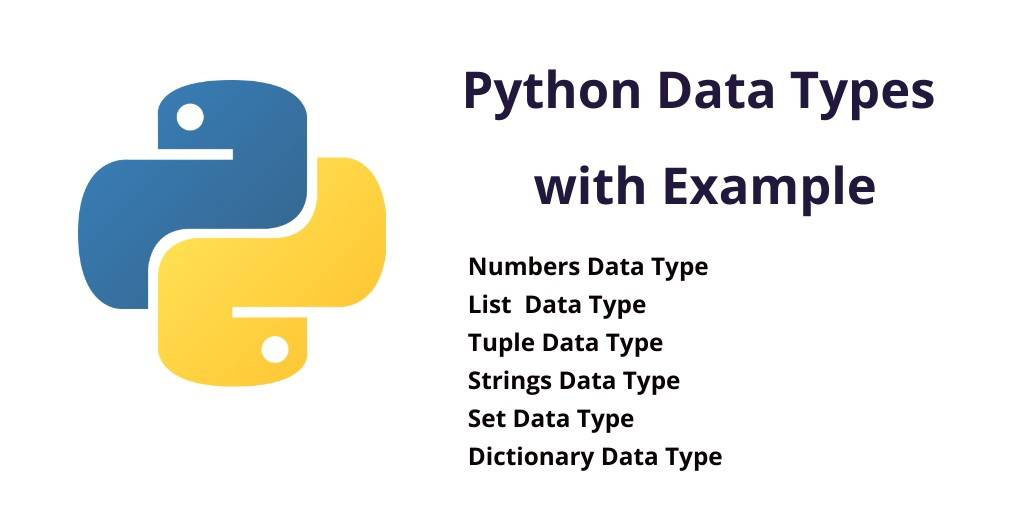Python 02-Jun-2022

### Python Data Types with Example

In this python data types tutorial, you will learn about python data types in detail with example

## Data Types in Python

### What is data types in python?

In Python programming language, a data type defines the type of a variable. Python programming does not require defining the data type of a variable. It is a dynamic type of language. The interpreter implicitly binds the value with its type.

### Types of data types in python

There are various data types in Python. Some of the important types are listed below:

• Numbers Data Type
• List Data Type
• Tuple Data Type
• Strings Data Type
• Set Data Type
• Dictionary Data Type

Note:- If you want to check the data type of a variable, what is the data type of the variable, then type () function is used to check the data type of a variable in Python.

In Python, below is the program for data type checking:

a = 'string'
b = 50
c = 10.5

print(type(a));
print(type(b));
print(type(c));

The output of the above program is following:

<type 'str'>
<type 'int'>
<type 'float'>

### Numbers Data Type

In python programming, Integer, floating-point numbers, and complex numbers under the category of Python numbers. Those you define as int, float and complex classes.

For example:

a = 10
print(a, "is of type", type(a))

a = 50.0
print(a, "is of type", type(a))

a = 11+2j
print(a, "is complex number?", isinstance(11+2j,complex))

Output of the above program is following:

10 is of type <class 'int'>
50.0 is of type <class 'float'>
(11+2j) is complex number? True

### List Data Type

In python programming, list data types hold different types of data. it does not need to be of the same type. The items stored in the list are separated with a comma (,) and enclosed within square brackets [].

Note:- List data types are alike to arrays in PHP, C

You can use a slicing operator [] to extract items from a list.

For example:

a = [10, 5.5, 'python']

print(a)

a = [5,10,15,20,25,30,35,40]

# a = 15
print("a = ", a)

# a[0:3] = [5, 10, 15]
print("a[0:3] = ", a[0:3])

# a[5:] = [30, 35, 40]
print("a[5:] = ", a[5:])

The output of the above program is following:

[10, 5.5, 'python']
a =  15
a[0:3] =  [5, 10, 15]
a[5:] =  [30, 35, 40]

In the above example, we have used the slicing operator [] to extract items or multiple items from a list. In Python, the index form starts at 0.

### Tuple Data Type

In python programming, a tuples data type is alike to list data type. But it is only one difference, once tuples created can not be changed/modify.

Tuples contain the collection of the items of different data types alike list data type. The items of the tuple are separated with a comma (,) and enclosed in parentheses ().

For example:

a = (8,'python', 1+2j)

# a = 'program'
print("a = ", a)

# a[0:3] = (5, 'program', (1+3j))
print("a[0:3] = ", a[0:3])

Output of the above code is following:

a =  python
a[0:3] =  (8, 'python', (1+2j))

### Strings Data Type

In python programming, A string is a sequence of characters in Python. In python, Strings are either enclosed with single quotes, double, and triple quotes.

Note:- In python, Multi-line strings can be denoted using triple quotes, ”’ or “””.

For example:

string = 'Hello world!'

# string = 'e'
print("string = ", string)

mString = """Hello world!
this is multi
line string example"""

print(mString)

Output of the above code is following:

string =  e
Hello world!
this is multi
line string example

### Set Data Type

In python programming, set data types hold unordered collection of unique items. The items stored in the set data types are separated with a comma (,) and enclosed within square brackets { }.

For example:

abc = {5,2,3,1,4}

# printing set variable
print("abc = ", abc)

# data type of variable a
print(type(abc))

Output of the above code is following:

abc =  {1, 2, 3, 4, 5}
<class 'set'>

### Dictionary Data Type

In python programming, Dictionary data types is held data in key and value pairs.

Note:- A dictionary data type does not allow duplicate keys in collection. But the values ??can be duplicate.

For example:

dict = {1:"john","lastname":"smith", "age":25}

# prints the value where key value is 1
print(dict)

# prints the value where key value is "lastname"
print(dict["lastname"])

# prints the value where key value is "age"
print(dict["age"])

Output of the above code is following:

john
smith
25